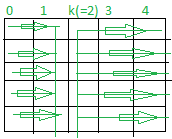Related Articles
Maximize the sum of Kth column of a Matrix
• Last Updated : 22 Sep, 2020

Given two integers N and K, the task is to maximize the sum of the Kth column of N * N row-wise sorted matrix consisting of element in the range [1, N2].

Examples:

Input: N = 2, K = 2
Output: {{1, 3}, {2, 4}}
Explanations: The possible row-wise sorted matrices are [{{1, 2}, {3, 4}}, {{1, 3}, {2, 4}}, {{1, 4}, {2, 3}}, {{3, 4}, {1, 2}}, {{2, 4}, {1, 3}}, {{2, 3}, {1, 4}} ]
Out of all the above possible matrices, the matrices [{{1, 3}, {2, 4}}, {{2, 4}, {1, 3}}, {{1, 4}, {2, 3}}, {{2, 3}, {1, 4}}] contains the maximum possible sum of the Kth column.
Therefore, one of the possible output is {{1, 3}, {2, 4}}.

Input: N = 3, K = 2
Output: {{1, 4, 5}, {2, 6, 7}, {3, 8, 9}}

Approach: The idea here is to first fill the indices smaller than the Kth columns of the matrix by the values from the range [1, N * (K – 1)] and then fill all the elements at columns greater than or equal to the kth column by values from the range [N * (K – 1) + 1, N2] as shown in the image below.Follow the steps below to solve the problem:

1. Fill all the columns of the matrix smaller than K by the values from the range [1, N * (K – 1)].
2. Then, fill the columns of the matrix greater than or equal to K by the values from the range [N * (K – 1) + 1, N * N].
3. Finally, print the matrix.

Below is the implementation of the above approach:

## C++

 `// C++ program to implement``// the above approach`` ` `#include ``using` `namespace` `std;`` ` `// Function to maximize the Kth column sum``int``** findMatrix(``int` `N, ``int` `K)``{`` ` `    ``// Store all the elements of the``    ``// resultant matrix of size N*N``    ``int``** mat = (``int``**)``malloc``(``        ``N * ``sizeof``(``int``*));`` ` `    ``for` `(``int` `i = 0; i < N; ++i) {``        ``mat[i] = (``int``*)``malloc``(``            ``N * ``sizeof``(``int``));``    ``}`` ` `    ``// Store value of each``    ``// elements of the matrix``    ``int` `element = 1;`` ` `    ``// Fill all the columns < K``    ``for` `(``int` `i = 0; i < N; ++i) {`` ` `        ``for` `(``int` `j = 0; j < K - 1; ++j) {``            ``mat[i][j] = element++;``        ``}``    ``}`` ` `    ``// Fill all the columns >= K``    ``for` `(``int` `i = 0; i < N; ++i) {`` ` `        ``for` `(``int` `j = K - 1; j < N; ++j) {``            ``mat[i][j] = element++;``        ``}``    ``}`` ` `    ``return` `mat;``}`` ` `// Function to print the matrix``void` `printMatrix(``int``** mat, ``int` `N)``{`` ` `    ``for` `(``int` `i = 0; i < N; ++i) {``        ``for` `(``int` `j = 0; j < N; ++j) {``            ``cout << mat[i][j] << ``" "``;``        ``}``        ``cout << endl;``    ``}``}`` ` `// Driver Code``int` `main()``{`` ` `    ``int` `N = 3, K = 2;``    ``int``** mat = findMatrix(N, K);`` ` `    ``printMatrix(mat, N);``}`

## Java

 `// Java program to implement``// the above approach``class` `GFG{`` ` `// Function to maximize the Kth column sum``static` `int` `[][]findMatrix(``int` `N, ``int` `K)``{`` ` `    ``// Store all the elements of the``    ``// resultant matrix of size N*N``    ``int` `[][]mat = ``new` `int``[N][N];`` ` `    ``// Store value of each``    ``// elements of the matrix``    ``int` `element = ``1``;`` ` `    ``// Fill all the columns < K``    ``for``(``int` `i = ``0``; i < N; ++i)``    ``{``        ``for``(``int` `j = ``0``; j < K - ``1``; ++j)``        ``{``            ``mat[i][j] = element++;``        ``}``    ``}`` ` `    ``// Fill all the columns >= K``    ``for``(``int` `i = ``0``; i < N; ++i) ``    ``{``        ``for``(``int` `j = K - ``1``; j < N; ++j)``        ``{``            ``mat[i][j] = element++;``        ``}``    ``}``    ``return` `mat;``}`` ` `// Function to print the matrix``static` `void` `printMatrix(``int` `[][]mat, ``int` `N)``{``    ``for``(``int` `i = ``0``; i < N; ++i) ``    ``{``        ``for``(``int` `j = ``0``; j < N; ++j)``        ``{``            ``System.out.print(mat[i][j] + ``" "``);``        ``}``        ``System.out.println();``    ``}``}`` ` `// Driver Code``public` `static` `void` `main(String[] args)``{``    ``int` `N = ``3``, K = ``2``;``    ``int` `[][]mat = findMatrix(N, K);`` ` `    ``printMatrix(mat, N);``}``}`` ` `// This code is contributed by Amit Katiyar`

## Python3

 `# Python3 program to implement``# the above approach`` ` `# Function to maximize the Kth ``# column sum``def` `findMatrix(N, K):``     ` `    ``# Store all the elements of the``    ``# resultant matrix of size N*N``    ``mat ``=` `[[``0` `for` `i ``in` `range``(N)]``              ``for` `j ``in` `range``(N)];`` ` `    ``# Store value of each``    ``# elements of the matrix``    ``element ``=` `0``;`` ` `    ``# Fill all the columns < K``    ``for` `i ``in` `range``(``0``, N):``        ``for` `j ``in` `range``(``0``, K ``-` `1``):``            ``element ``+``=` `1``;``            ``mat[i][j] ``=` `element;`` ` `    ``# Fill all the columns >= K``    ``for` `i ``in` `range``(``0``, N):``        ``for` `j ``in` `range``(K ``-` `1``, N):``            ``element ``+``=` `1``;``            ``mat[i][j] ``=` `element;`` ` `    ``return` `mat;`` ` `# Function to prthe matrix``def` `printMatrix(mat, N):``     ` `    ``for` `i ``in` `range``(``0``, N):``        ``for` `j ``in` `range``(``0``, N):``            ``print``(mat[i][j], end ``=` `" "``);`` ` `        ``print``();`` ` `# Driver Code``if` `__name__ ``=``=` `'__main__'``:``     ` `    ``N ``=` `3``; K ``=` `2``;``    ``mat ``=` `findMatrix(N, K);`` ` `    ``printMatrix(mat, N);`` ` `# This code is contributed by Amit Katiyar`

## C#

 `// C# program to implement``// the above approach``using` `System;`` ` `class` `GFG{`` ` `// Function to maximize the Kth column sum``static` `int` `[,]findMatrix(``int` `N, ``int` `K)``{`` ` `    ``// Store all the elements of the``    ``// resultant matrix of size N*N``    ``int` `[,]mat = ``new` `int``[N, N];`` ` `    ``// Store value of each``    ``// elements of the matrix``    ``int` `element = 1;`` ` `    ``// Fill all the columns < K``    ``for``(``int` `i = 0; i < N; ++i)``    ``{``        ``for``(``int` `j = 0; j < K - 1; ++j)``        ``{``            ``mat[i, j] = element++;``        ``}``    ``}`` ` `    ``// Fill all the columns >= K``    ``for``(``int` `i = 0; i < N; ++i) ``    ``{``        ``for``(``int` `j = K - 1; j < N; ++j)``        ``{``            ``mat[i, j] = element++;``        ``}``    ``}``    ``return` `mat;``}`` ` `// Function to print the matrix``static` `void` `printMatrix(``int` `[,]mat, ``int` `N)``{``    ``for``(``int` `i = 0; i < N; ++i) ``    ``{``        ``for``(``int` `j = 0; j < N; ++j)``        ``{``            ``Console.Write(mat[i, j] + ``" "``);``        ``}``        ``Console.WriteLine();``    ``}``}`` ` `// Driver Code``public` `static` `void` `Main(String[] args)``{``    ``int` `N = 3, K = 2;``    ``int` `[,]mat = findMatrix(N, K);`` ` `    ``printMatrix(mat, N);``}``}`` ` `// This code is contributed by Amit Katiyar`
Output:
```1 4 5
2 6 7
3 8 9
```

Time Complexity: O(N2)
Auxiliary Space: O(N2)

Attention reader! Don’t stop learning now. Get hold of all the important DSA concepts with the DSA Self Paced Course at a student-friendly price and become industry ready.

My Personal Notes arrow_drop_up